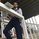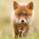1896 visualizzazioni
1896
Moving average slope and momentum Analysis, dark color its speeding up, light color slowing
```study(title="[RS]Trend Slope Signal", shorttitle="[RS]TSS")

//  ||---   Inputs:
length1 = input(4)
length2 = input(8)
length3 = input(16)
length4 = input(24)
length5 = input(48)
length6 = input(72)
length7 = input(120)
length8 = input(240)
length9 = input(576)
//  ||---   Moving Averages:
ma1 = ema(close, length1)
ma2 = ema(close, length2)
ma3 = ema(close, length3)
ma4 = ema(close, length4)
ma5 = ema(close, length5)
ma6 = ema(close, length6)
ma7 = ema(close, length7)
ma8 = ema(close, length8)
ma9 = ema(close, length9)

//  ||--- Is slope rising or falling ?
slope1 = ma1 > ma1
slope2 = ma2 > ma2
slope3 = ma3 > ma3
slope4 = ma4 > ma4
slope5 = ma5 > ma5
slope6 = ma6 > ma6
slope7 = ma7 > ma7
slope8 = ma8 > ma8
slope9 = ma9 > ma9
//  ||--- Is Slope gaining momentum?
mom1 = (ma1 - ma1) < (ma1 - ma1)
mom2 = (ma2 - ma2) < (ma2 - ma2)
mom3 = (ma3 - ma3) < (ma3 - ma3)
mom4 = (ma4 - ma4) < (ma4 - ma4)
mom5 = (ma5 - ma5) < (ma5 - ma5)
mom6 = (ma6 - ma6) < (ma6 - ma6)
mom7 = (ma7 - ma7) < (ma7 - ma7)
mom8 = (ma8 - ma8) < (ma8 - ma8)
mom9 = (ma9 - ma9) < (ma9 - ma9)
//  ||--- Conditional coloring:
optionalred = #b26666   // Fading signal color
optionallime = #66b266  // Fading signal color
color1 = slope1 and mom1 ? green : slope1 and (not mom1) ? optionallime :
not slope1 and mom1 ? maroon : (not slope1) and (not mom1) ? optionalred : na

color2 = slope2 and mom2 ? green : slope2 and (not mom2) ? optionallime :
not slope2 and mom2 ? maroon : (not slope2) and (not mom2) ? optionalred : na

color3 = slope3 and mom3 ? green : slope3 and (not mom3) ? optionallime :
not slope3 and mom3 ? maroon : (not slope3) and (not mom3) ? optionalred : na

color4 = slope4 and mom4 ? green : slope4 and (not mom4) ? optionallime :
not slope4 and mom4 ? maroon : (not slope4) and (not mom4) ? optionalred : na

color5 = slope5 and mom5 ? green : slope5 and (not mom5) ? optionallime :
not slope5 and mom5 ? maroon : (not slope5) and (not mom5) ? optionalred : na

color6 = slope6 and mom6 ? green : slope6 and (not mom6) ? optionallime :
not slope6 and mom6 ? maroon : (not slope6) and (not mom6) ? optionalred : na

color7 = slope7 and mom7 ? green : slope7 and (not mom7) ? optionallime :
not slope7 and mom7 ? maroon : (not slope7) and (not mom7) ? optionalred : na

color8 = slope8 and mom8 ? green : slope8 and (not mom8) ? optionallime :
not slope8 and mom8 ? maroon : (not slope8) and (not mom8) ? optionalred : na

color9 = slope9 and mom9 ? green : slope9 and (not mom9) ? optionallime :
not slope9 and mom9 ? maroon : (not slope9) and (not mom9) ? optionalred : na

plot(0, color=black, linewidth=1)
plot(3, color=color1, linewidth=10)
plot(3.5, color=color2, linewidth=10)
plot(4, color=color3, linewidth=10)
plot(4.5, color=color4, linewidth=10)
plot(5, color=color5, linewidth=10)
plot(5.5, color=color6, linewidth=10)
plot(6, color=color7, linewidth=10)
plot(6.5, color=color8, linewidth=10)
plot(7, color=color9, linewidth=10)
plot(10, color=black, linewidth=1)
```Is there any way to stop the re-painting of the previous bar?
Rispondiyes it looks to be when you zoom out enought 1 in 2 ticks(bars) disapear, try to zoom in and see if problem persists?
Rispondihi and thanks for the comments, it may be a overlay from using the thick lines over the advisable limit pixelated effect, either way i will look into it.
Rispondi